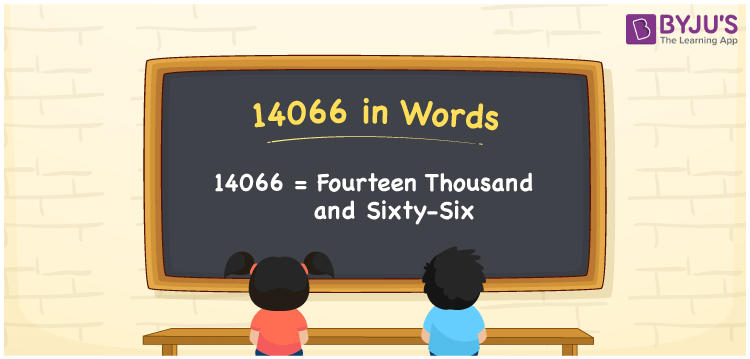We can write 14066 in words as Fourteen thousand and sixty-six. Suppose you made a digital transaction of Rs. 14066, then you can say, “I made a digital transaction of Fourteen thousand and sixty-six rupees”. Here, 14066 is the cardinal number since it shows the specific amount, and Fourteen thousand and sixty-six is the number name of 14066.

 14066 in words Fourteen thousand and sixty-six Fourteen thousand and sixty-six in Numbers 14066

## 14066 in English Words

In general, we use the English alphabet to write numbers in words. So, we spell 14066 in English as “Fourteen thousand and sixty-six”.## How to Write 14066 in Words?

As we can see, 14066 is a five-digit number, so we need to create a place value chart in such a way that all the digits of 14066 are defined with the respective place value. This can be done as follows.

 Ten thousand Thousands Hundreds Tens Ones 1 4 0 6 6

Here, ones = 6, tens = 6, hundreds = 0, thousands = 4, ten thousand = 1

These can be written as:

1 × Ten thousand + 4 × Thousand + 0 × Hundred + 6 × Ten + 6 × One

= 1 × 10000 + 4 × 1000 + 0 × 100 + 8 × 10 + 0 × 1

= 10000 + 4000 + 60 + 6

= Ten Thousand + Four thousand + Sixty + Six

= Fourteen thousand + Sixty-six

= Fourteen thousand and sixty-six

Therefore, 14066 in words = Fourteen thousand and sixty-six.

14066 is a natural number that is the successor of 14065 and predecessor of 14067.

14066 in words – Fourteen thousand and sixty-six

Is 14066 an odd number? – No

Is 14066 an even number? – Yes

Is 14066 a prime number? – No

Is 14066 a composite number? – Yes

Is 14066 a perfect square number? – No

Is 14066 a perfect cube number? – No

## Frequently Asked Questions on 14066 in Words

Q1

### How do you write 14066 in words?

We can write the number 14066 in words as Fourteen thousand and sixty-six.
Q2

### What is 14066 in words?

Fourteen thousand and sixty-six is 14066 in words.
Q3

### How to write an amount of Rs. 14066 in words on a cheque?

On a cheque, we can express an amount of Rs. 14066 in words as “Fourteen thousand and sixty-six rupees only”.